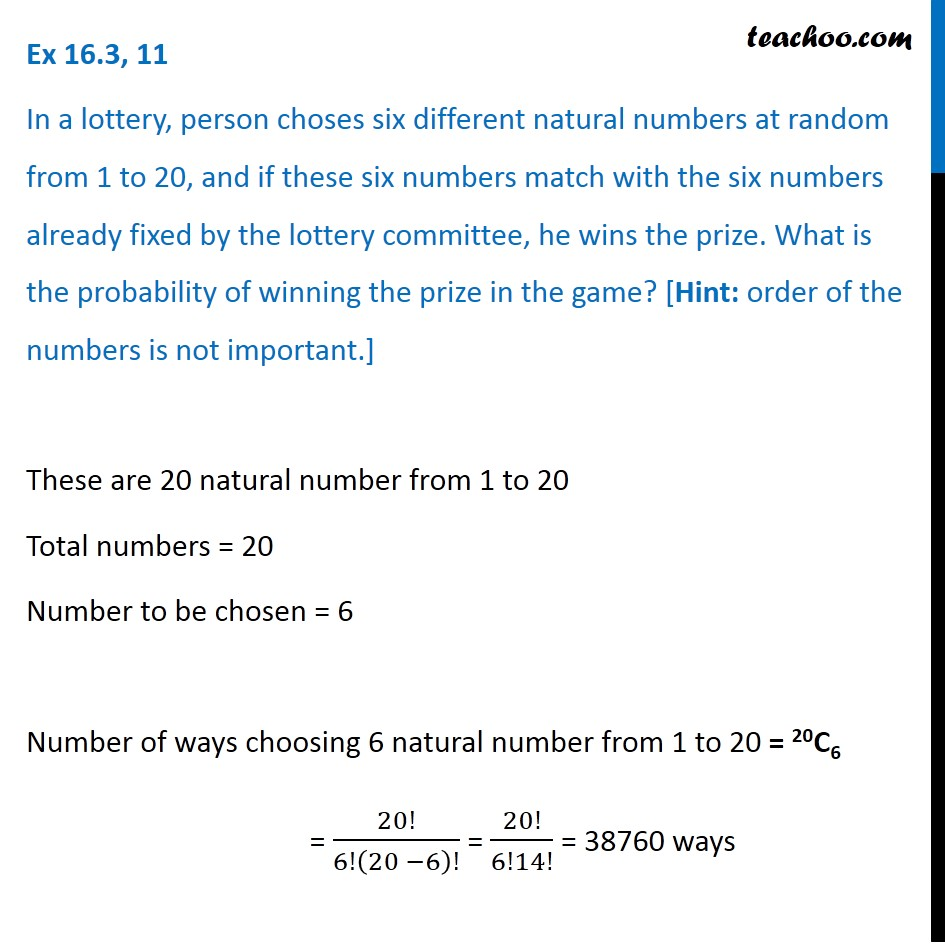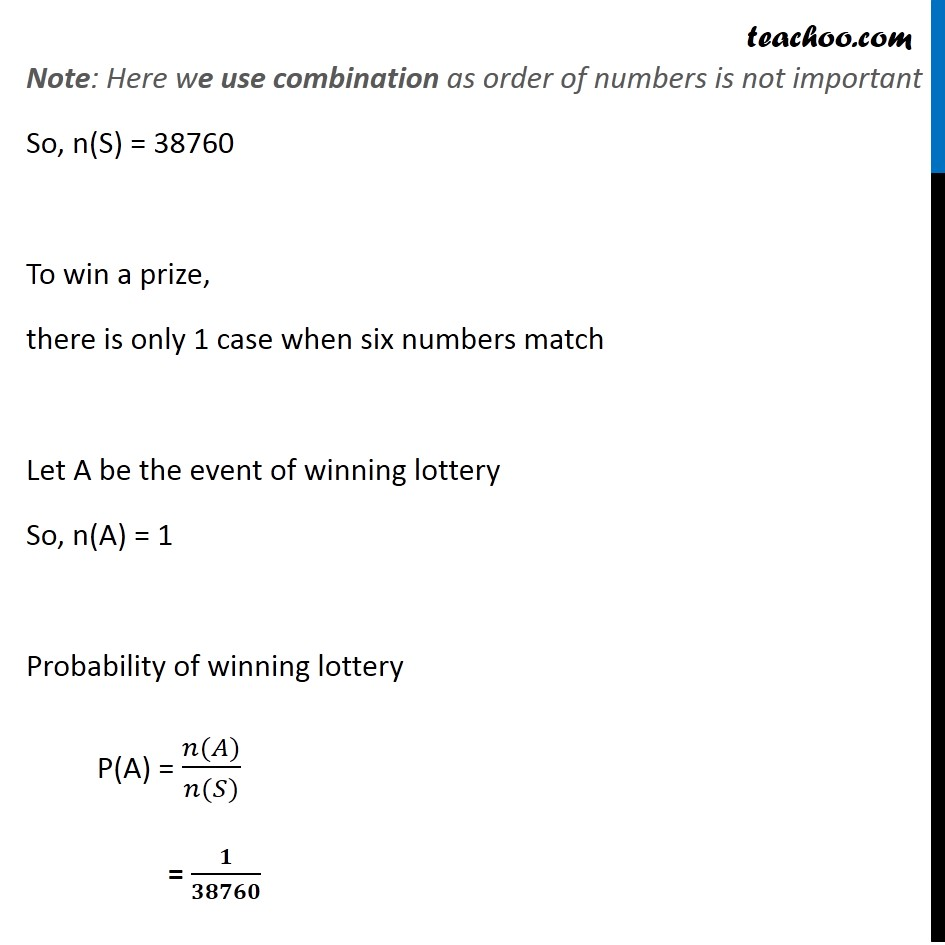Using combination

Chapter 14 Class 11 Probability
Concept wiseLearn in your speed, with individual attention - Teachoo Maths 1-on-1 Class

### Transcript

Ex 14.2, 11 In a lottery, person choses six different natural numbers at random from 1 to 20, and if these six numbers match with the six numbers already fixed by the lottery committee, he wins the prize. What is the probability of winning the prize in the game? [Hint: order of the numbers is not important.] These are 20 natural number from 1 to 20 Total numbers = 20 Number to be chosen = 6 Number of ways choosing 6 natural number from 1 to 20 = 20C6 = 20!/6!(20 −6)! = 20!/6!14! = 38760 ways Note: Here we use combination as order of numbers is not important So, n(S) = 38760 To win a prize, there is only 1 case when six numbers match Let A be the event of winning lottery So, n(A) = 1 Probability of winning lottery P(A) = (𝑛(𝐴))/(𝑛(𝑆)) = 𝟏/𝟑𝟖𝟕𝟔𝟎